Function Repository Resource:

# PointsToRules

Convert a list of points into a list of substitution rules

Contributed by: Dennis M Schneider
 ResourceFunction["PointsToRules"][pts,vars] converts the list of pts into a list of substitution rules in vars.

## Examples

### Basic Examples (4)

Convert a list of numbers into a list of substitution rules:

 In:=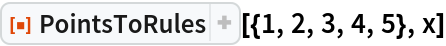Out=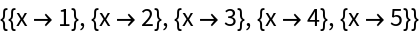This is the same as:

 In:=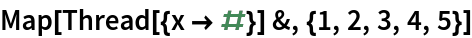Out=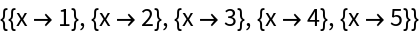Convert a list of n-tuples into a list of substitution rules in n variables:

 In:=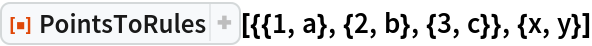Out=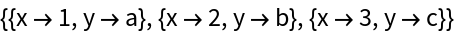This is the same as:

 In:=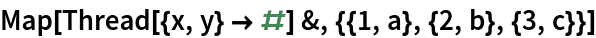Out=Convert an n-tuples into a list of substitution rules in n variables:

 In:=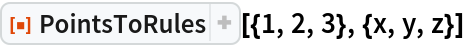Out=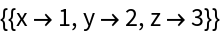This is the same as:

 In:=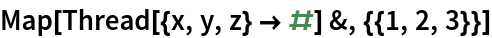Out=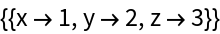Convert a list of n-tuples into a list of associations using the resource function ToAssociations:

 In:=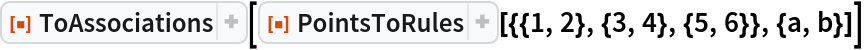Out=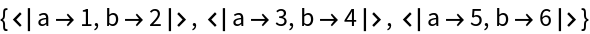### Scope (3)

The number of variables must be compatible with the size of each point:

 In:=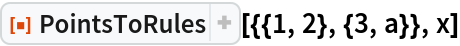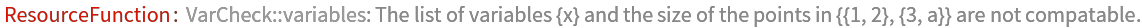In:=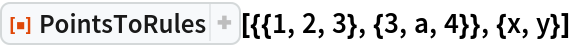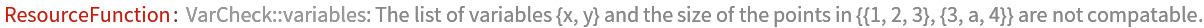The first argument must be a vector or a matrix:

 In:=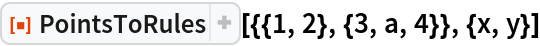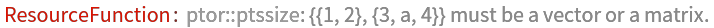The second argument must be a symbol or a list of symbols:

 In:=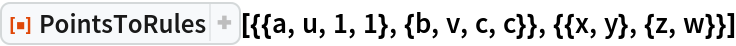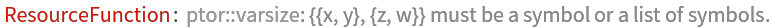## Publisher

Dennis M Schneider

## Version History

• 3.0.0 – 04 November 2019
• 2.0.0 – 11 October 2019
• 1.0.0 – 08 August 2019